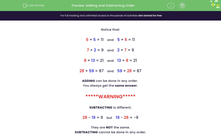### Comprehensive & curriculum aligned

In this worksheet, students must understand that addition can be done in any order but subtraction cannot.Key stage:  KS 1

Curriculum topic:   Number: Addition and Subtraction

Curriculum subtopic:   Understand Order for Addition/Subtraction

Popular topics:   Subtraction worksheets, Addition worksheets

Difficulty level:#### Worksheet Overview

Notice that:

6 + 5 = 11    and    5 + 6 = 11

7 + 2 = 9    and    2 + 7 = 9

8 + 13 = 21    and    13 + 8 = 21

28 + 59 = 87    and    59 + 28 = 87

ADDING can be done in any order.

You always get the same answer.

*****WARNING*****

SUBTRACTING is different.

28 - 19 = 9    but    19 - 28 = -9

They are NOT the same.

SUBTRACTING cannot be done in any order.

### What is EdPlace?

We're your National Curriculum aligned online education content provider helping each child succeed in English, maths and science from year 1 to GCSE. With an EdPlace account you’ll be able to track and measure progress, helping each child achieve their best. We build confidence and attainment by personalising each child’s learning at a level that suits them.

Get started#### Popular Maths topics

••••